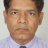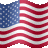# Combining Custom indicator + PPS in a backtest?

M

#### mynameisdavid67

##### New member
If this along with the PPS buy signal are true within 3-5 bars, on either a one or five minute time frame. Buy. If price opens and closes below the 9 SMA, sell. Only to take long positions.

Code:
``````declare lower;
input fastLength = 12;
input slowLength = 26;
input MACDLength = 9;
input averageTypeMACD = AverageType.EXPONENTIAL;

def Diff = MACD(fastLength, slowLength, MACDLength, averageTypeMACD).Diff;

input length = 7;
input price = close;
input averageType = AverageType.WILDERS;

def NetChgAvg = MovingAverage(averageType, price - price, length);
def TotChgAvg = MovingAverage(averageType, AbsValue(price - price), length);
def ChgRatio = if TotChgAvg != 0 then NetChgAvg / TotChgAvg else 0;

def RSI = 50 * (ChgRatio + 1);

def twoBarPivotMACD = Diff > Diff and Diff > Diff and Diff < Diff and Diff < Diff;

def overSoldRSI = RSI <= 30;

plot TrendReversal = twoBarPivotMACD and Highest(overSoldRSI, 2) > 0;``````

Last edited by a moderator:Exploring Scan Combining Unusual volume and near 52 weeks high ... Playground 26Combining Lower Indicators on One Window? Questions 8